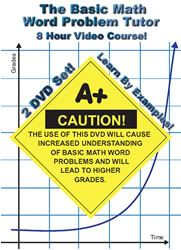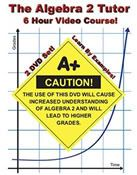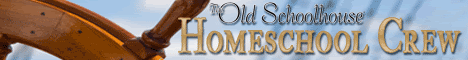"Math Tutor DVD offers Math Help via DVD video tutorial content." The instructor, Jason Gibson, holds Master’s degrees in both Electrical Engineering and Physics. I received 2 courses from the Math Tutor DVD to review. The first course is Basic Math Word Problems. There are 8 hours of video covering the following topics.Disc 1 Section 1: Adding Whole Numbers Section 2: Subtracting Whole Numbers Section 3: Multiplying Whole Numbers Section 4: Dividing Whole Numbers Section 5: Adding Decimals Section 6: Subtracting Decimals Section 7: Multiplying Decimals Section 8: Dividing Decimals Disc 2 Section 9: Adding Fractions Section 10: Subtracting Fractions Section 11: Multiplying Fractions Section 12: Dividing Fractions Section 13: Percents, Part 1 Section 14: Percents, Part 2 Section 15: Ratio and Proportion

I also received the Algebra 2 Tutor which is a 6 hour course covering the following topics.Disk 1 Section 1: Graphing Equations Section 2: The Slope Of A Line Section 3: Writing Equations Of Lines Section 4: Graphing Inequalities Section 5: Solving Systems Of Equations By Graphing Section 6: Solving Systems Of Equations By Substitution Section 7: Solving Systems Of Equations By Addition Disk 2 Section 8: Solving Systems Of Equations In Three Variables Section 9: Simplifying Radical Expressions. Section 10: Add/Subtract Radical Expressions. Section 11: Multiply/Divide Radical Expressions. Section 12: Solving Equations With Radicals. Section 13: Fractional Exponents. Section 14: Solving Polynomial Equations. Section 15: The Quadratic Formula.

Both of the courses are similar in their presentation. First the instructor offers a very brief introduction to the topic of each section. He then proceeds to work example problems pertaining to the topic.  He works the problems very deliberately, without skipping steps, so that the the student can easily keep up with him. (There are times where I think he uses too many steps, notably in problems involving multiplication by a zero.)  The visual presentation is very simple and consists only of the instructor, a whiteboard, and dry erase markers.

I was impressed by several aspects of the videos.

• The instructor has a firm grasp of the concepts he is teaching.  This was especially evident in the Algebra 2 course, since it is a subject that is more complicated.  I found his explanations easy to follow, and was reminded of my Algebra 2 teacher with his method of making a chart of x and y coordinates, and determining the curve by plotting the points. (I loved my Algebra teacher so I meant that as a good thing!)
• I liked how he points out key words to look for when deciding which mathematical operation to use in a word problem.
• I also liked that he introduces the concept of canceling units in the basic word problems course.  That is a concept that I don’t remember being taught until college, and it was a light bulb moment when I saw that units could be used to help determine how to solve the problem. (My husband assures me that he teaches this concept to his high school chemistry class, but I don’t remember learning it in high school.)
• I also appreciate the simplicity of the presentation.  Often too much effort is spent in trying to make things flashy and exciting, while the concept that is supposedly being taught is weak.
• The DVDs are very reasonably priced for their length.  These courses are currently only \$26.99 each.

Keep in mind that these courses are designed to serve as extra help when a student is struggling with their regular curriculum. I do not have a student that is struggling in Math Word Problems, nor do I have a student who is taking Algebra 2.  Because of this, I cannot give any testimony to the effectiveness of this product.  However, the company offers a full money-back guarantee, if you are not fully satisfied.

Math Tutor DVD offers many additional courses including 3 levels of Calculus and 2 levels of Physics. You can view samples of each of the courses at their website www.MathTutorDVD.com

You can read other reviews of the Math Tutor DVD at the TOS Homeschool Crew Blog .By Kristen H.
1.Anonymous says: